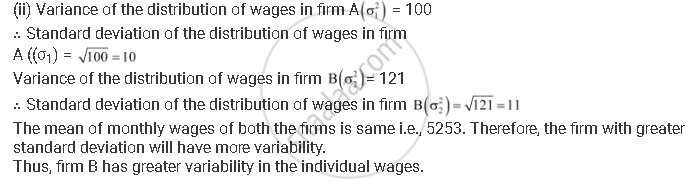CBSE (Arts) Class 11CBSE
Share

# An Analysis of Monthly Wages Paid to Workers in Two Firms a and B, Belonging to the Same Industry, Gives the Following Results::Which Firm a Or B Pays Larger Amount as Monthly Wages? - CBSE (Arts) Class 11 - Mathematics

ConceptVariance and Standard Deviation Shortcut Method to Find Variance and Standard Deviation

#### Question

An analysis of monthly wages paid to workers in two firms A and B, belonging to the same industry, gives the following results::

 Firm A Firm B No. of wage earners 586 648 Mean of monthly wages Rs 5253 Rs 5253 Variance of the distribution of wages 100 121

(i) Which firm A or B pays larger amount as monthly wages?

(ii) Which firm, A or B, shows greater variability in individual wages?

#### Solution

(i) Monthly wages of firm A = Rs 5253

Number of wage earners in firm A = 586

∴Total amount paid = Rs 5253 × 586

Monthly wages of firm B = Rs 5253

Number of wage earners in firm B = 648

∴Total amount paid = Rs 5253 × 648

Thus, firm B pays the larger amount as monthly wages as the number of wage earners in firm B are more than the number of wage earners in firm A.Is there an error in this question or solution?

#### Video TutorialsVIEW ALL 

Solution An Analysis of Monthly Wages Paid to Workers in Two Firms a and B, Belonging to the Same Industry, Gives the Following Results::Which Firm a Or B Pays Larger Amount as Monthly Wages? Concept: Variance and Standard Deviation - Shortcut Method to Find Variance and Standard Deviation.
S